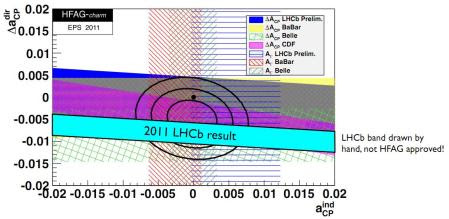## Monday, November 14, 2011

### LHCb reports a new source of CP-violation

Well, just another 3-sigma hint that may go away

Mat Charles of Oxford gave a talk at HCP 2011 in Paris in which he reported a surprising result by LHCb at CERN based on 0.58/fb of their 2011 data.

Some CP-violating asymmetries $$A_{CP}$$ are measured for the decays
$D^0 \to K^+ K^-, \quad D^0 \to \pi^+\pi^-.$ The asymmetries are defined as the ratio of difference and the sum of the partial decay rates for decays of the particle or its antiparticle to the other mesons:
$A_{CP}^{\pi{\rm \,\,or\,\,} K} = \frac{\Gamma(D^0\to f^+ f^-)-\Gamma(\bar D^0\to f^+ f^-)}{\Gamma(D^0\to f^+ f^-)+\Gamma(\bar D^0\to f^+ f^-)}$ where $$f^\pm=\pi^\pm$$ or $$K^\pm$$. This asymmetry is a "direct way" to access the CP-violation by particle collisions; an indirect way would be to look at the $$D^0$$-$$\bar D^0$$ oscillations.

The difference between these two asymmetries $$\Delta A_{CP}=A_{CP}^{\pi} - A_{CP}^K$$ computed for the decay channels of the charmed meson should be (almost but not quite exactly) zero according to the Standard Model (assuming that one properly adjusts the signs: I don't quite follow them right now): the only source of the CP-violation is the CKM complex phase.

However, the measured difference is
$\Delta A_{CP} = -0.82\% \pm 0.24\%$ which is roughly 3.4 standard deviations away from the prediction. The rumors described it as 3.5 sigma which was a slight overestimate. In the error, $$0.21\%$$ is statistical and $$0.11\%$$ is systematic (they were added in quadrature).The discrepancy is visualized by the fact that the black theoretical SM dot at "zero" isn't sitting within the 1-sigma cyan experimental LHCb band.

Whether the deviation is a human error, a fluke, or a sign of new physics remains to be seen.